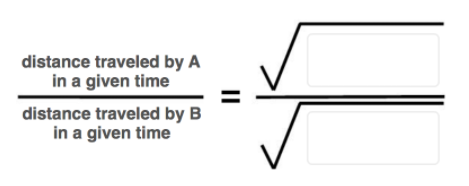# Problem: Complete each of the following equations regarding diffusion of two gases, A and B.

###### FREE Expert Solution

We’re being asked to set up the equation for diffusion

Recall that Graham's Law of Effusion allows us to compare the rate of effusion of two gases. Graham's Law states that the rate of effusion of a gas is inversely proportional to its molar mass.

89% (124 ratings)###### Problem Details

Complete each of the following equations regarding diffusion of two gases, A and B.